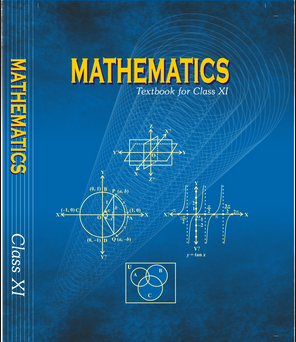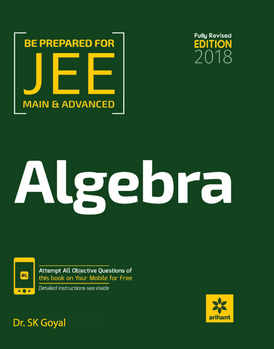# Sequence and series (Weightage 5%)   Share

### Topics from Sequence and series

• Arithmetic and geometric progressions,insertion of arithmetic (32 concepts)
• Geometric means between two given numbers (2 concepts)
• Arithmetic, Geometric & Harmonic Progressions (7 concepts)
• Relation between A.M. and G.M. (3 concepts)
• Sum upto n terms of special series: Sn, Sn2, Sn3 (7 concepts)
• Sequences, Series And Progression (1 concepts)
• Arithmetic Progression (6 concepts)
• Geometric Progression (9 concepts)
• Harmonic Progression (3 concepts)
• Arithmetico-Geometric Progression (3 concepts)
• Application Of A.M.-G.M. (3 concepts)
• Summation By Sigma(?) Operator (4 concepts)
• Method Of Differences (3 concepts)
• Sum of Some Special Series (Vn Method) (2 concepts)

### Important Books for Sequence and series

••Exams
Articles
Questions